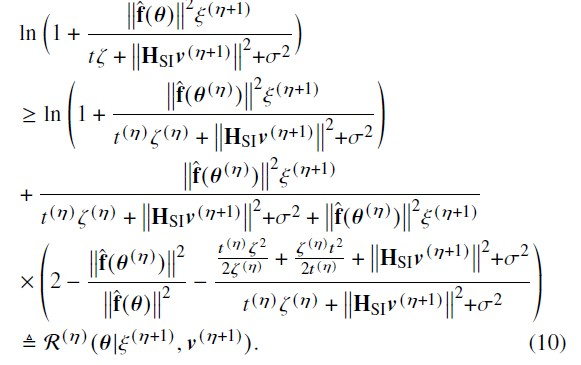# How to write 1/norm(x)^2 in cvx?

My function is 1/norm(f_theta)^2;
Where f_theta = f + a_u*diag(h)*phase_shift; Here, phase_shift is the optimization variable.

And cvx is accepting following command:

inv_pos(square_pos(norm(f_theta_opt))).

The objective function is as follows:And the corresponding MATLAB code is as follows:

obj_4 = log ( 1 + ( (f_theta’f_theta)P_UE_inc) / ( t_initialx_initial …
+ square_pos(norm(h_s_i
Tx_beamforming_inc)) + sigma_sqr ) );
obj_5 = ( (f_theta’f_theta)P_UE_inc) / ( t_initialx_initial + …
square_pos(norm(h_s_i
Tx_beamforming_inc)) + sigma_sqr + (f_theta’*f_theta)*P_UE_inc ) ;

``````       obj_6 = ( ( (t_initial*square_pos(x))/(2*x_initial) ) + ((x_initial*square_pos(t))/(2*t_initial)) ...
+ (square_pos(norm(h_s_i*Tx_beamforming_inc))) + (sigma_sqr) ) / (t_initial*x_initial ...
+ square_pos(norm(h_s_i*Tx_beamforming_inc))  + sigma_sqr  )  ;

obj_7 = obj_5*( 2 - (f_theta'*f_theta).*inv_pos(square_pos(norm(f_theta_opt))) - obj_6) ;

obj_8 =  obj_4 + obj_7; % Equation (10)
``````

Error is given as follows:

Error using cvx/pow_cvx
Disciplined convex programming error:
Illegal operation: pow_p( {convex}, {-1} )

Error in cvx/inv_pos (line 5)
y = pow_cvx( x, -1, ‘pow_p’ );

Error in convergence_beam_main_1 (line 319)
obj_7 = obj_5*( 2 - (f_theta’*f_theta).*inv_pos(square_pos(norm(f_theta_opt))) - obj_6) ;

1/\|x\|^2 or any similar inverse-norms are both non-convex and non-concave so they cannot be used in any context in CVX.

Using `inv_pos(-square_pos(norm(f_theta_opt)))` implies `f_theta_opt==0`. That’s a very weird construct.

The function 1/∥x∥^2 , where x is 5X1 matrix size.
According to your suggestions, it can not be implemented in CVX Tool? Am I right Sir.

But, the function is convex and before that -m sign is there, hence it is concave (Already given explanation).

Shall I look for any other tool for this optimization?

If f is convex it does not immediately imply that 1/f is concave (nor convex). The function 1/\|x\|^2 is neither. Maybe you need some other tool or another reformulation.

Ok Sir.
Thank you for the information.